At Browserling we're building a network of online tools websites. Each site focuses on one and only one tool category. Each tool does one and only one thing.

Today we're releasing the eighteenth site – Online Fractal Tools.

Online Fractal Tools is a collection of simple, free and easy to use utilities for doing all kinds of fractaly things. There are no ads, popups or other garbage. Just fractal utilities that work in your browser. Press a button – get a fractal!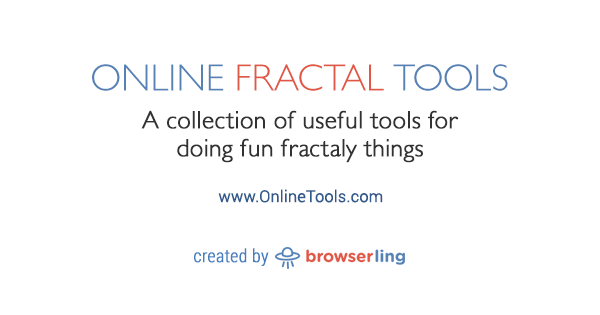www.onlineFRACTALtools.com

Here's a list of all fractal tools so far.

Draw a Dendrite Fractal that looks like this: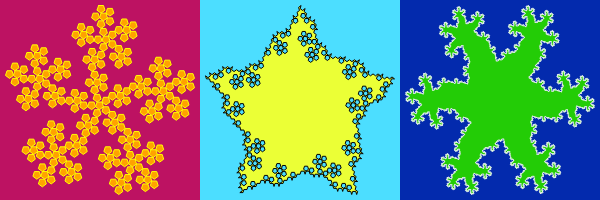Draw a Canopy Fractal that looks like this: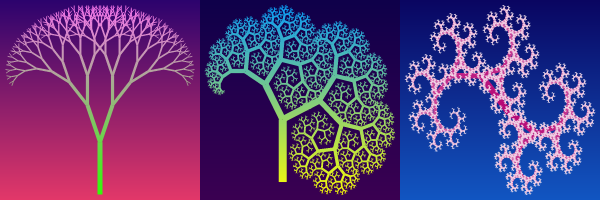Draw a Flowsnake Fractal that looks like this: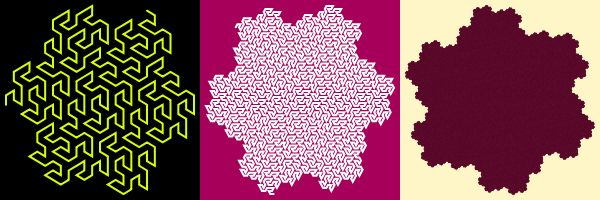Draw a Morton Fractal that looks like this: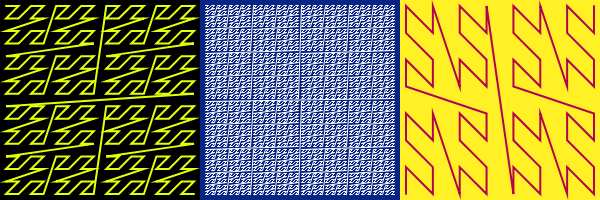Draw a Hilbert Fractal that looks like this: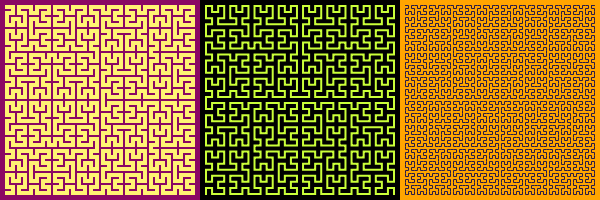Draw a V-fractal that looks like this: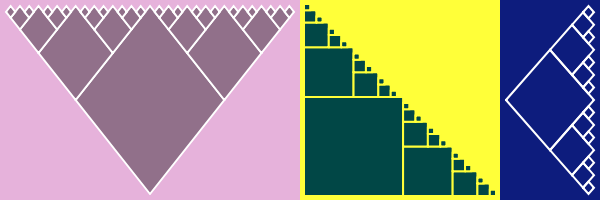Draw a Peano Fractal that looks like this: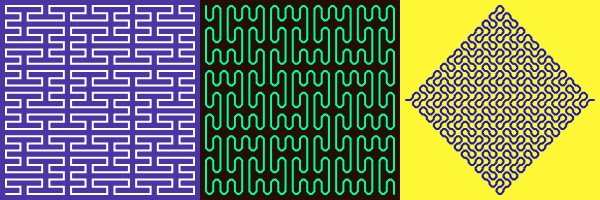Draw a Dragon Fractal that looks like this: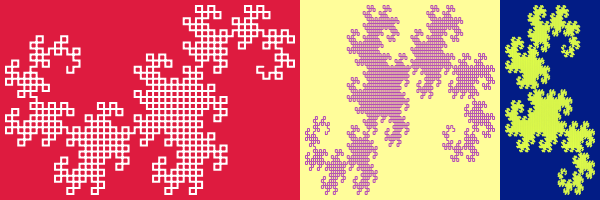Draw a Twin Dragon Fractal that looks like this: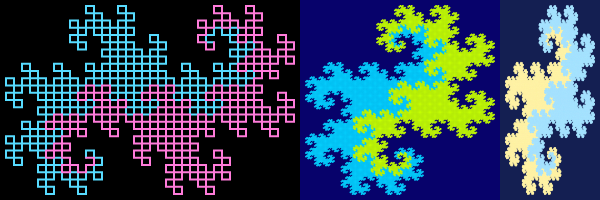Draw a Triangle Dragon Fractal that looks like this: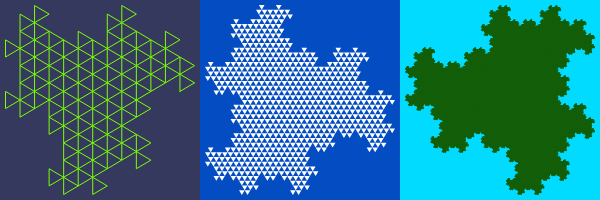Draw a Koch Fractal that looks like this: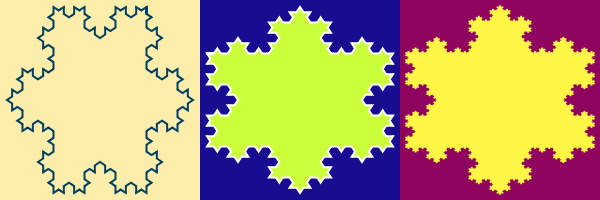Draw a Triflake Fractal that looks like this: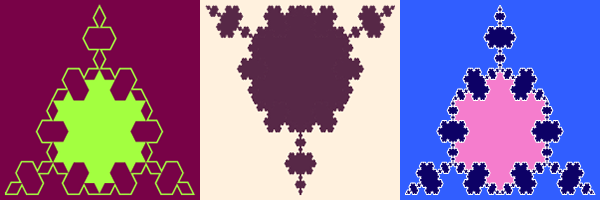Draw a Sierpinski Fractal that looks like this: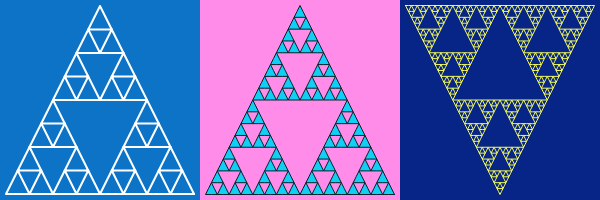Draw a Pentaflake Fractal that looks like this: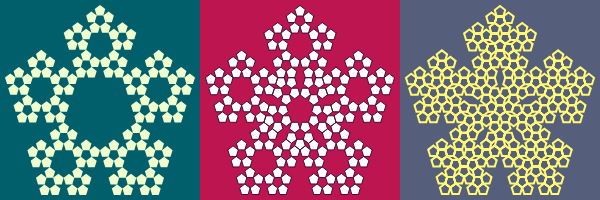Draw a Hexaflake Fractal that looks like this: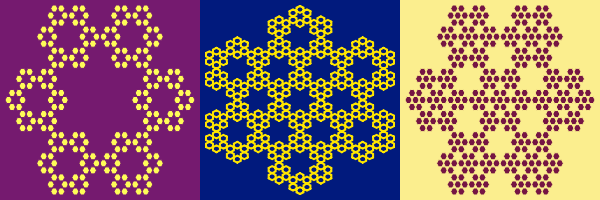Draw a Multiflake Fractal that looks like this: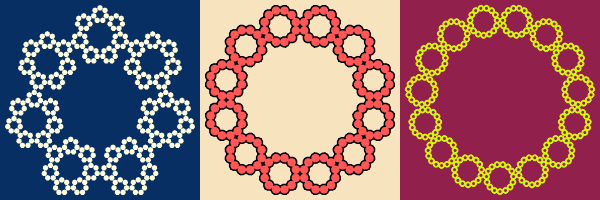Draw a Moore Fractal that looks like this: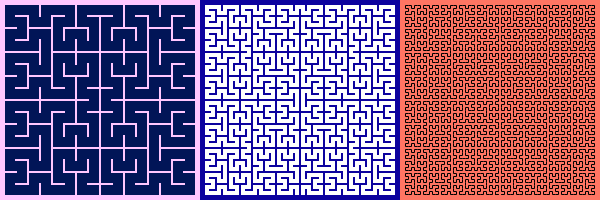Draw a Cantor Fractal that looks like this:Draw a Dust Fractal that looks like this: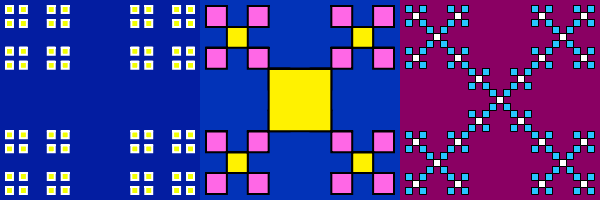Draw a Levy Fractal that looks like this: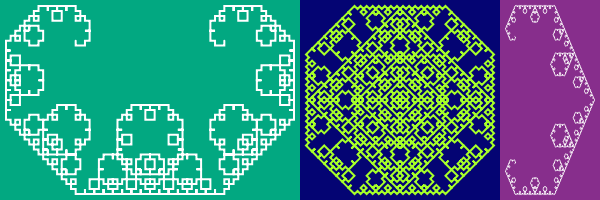Draw a Frosty Fractal that looks like this: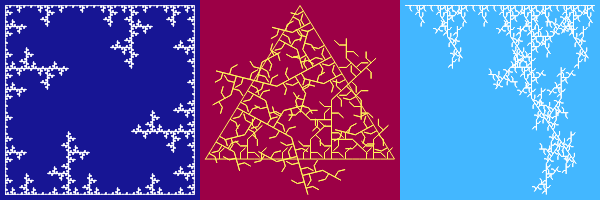Draw a Pythagoras Fractal that looks like this: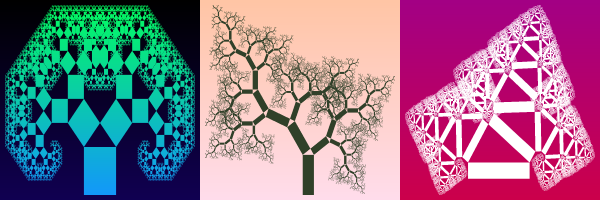Draw a T-square Fractal that looks like this: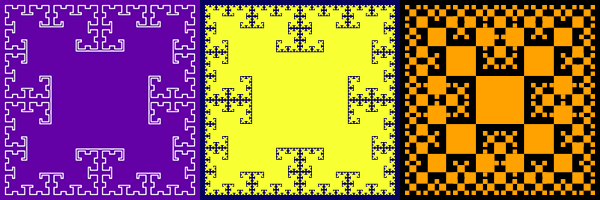Draw a Hausdorff Fractal that looks like this: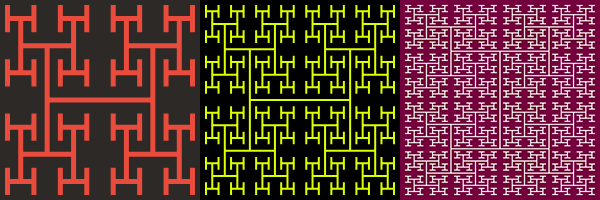Here are the upcoming fractal tools:

• Generate a Hilbert Sequence
• Enumerate a Hilbert Fractal
• Generate a Peano Sequence
• Enumerate a Peano Fractal
• Generate a Moore Sequence
• Enumerate a Moore Sequence
• Generate a Cantor String
• Draw a Mandelbrot Tree
• Draw a Barnsey Tree
• Draw a Barnsey's Fern
• Draw a Binary Fractal Tree
• Draw a Ternary Fractal Tree
• Draw a Dragon Fractal Tree
• Draw a De Rham Fractal
• Draw a Takagi Fractal
• Draw a Peano Pentagon Fractal
• Draw a Tridendrite Fractal
• Draw a McWorter Pentigree Fractal
• Draw a McWorter Lucky Seven Fractal
• Draw an Eisenstein Fractions Fractal
• Draw a Bagula Double V Fractal
• Draw a Julia Set
• Draw a Mandelbrot Set
• Draw a Toothpick Fractal
• Draw a Ulam-Warburton Fractal

The first seventeen websites in the network are:

The next few sites are onlineHASHtools, onlineGIFtools, onlineBMPtools, onlinePDFtools, onlineBROWSERtools, onlineCRYPTOtools, onlineAUDIOtools, onlineCSStools, onlineJStools, and then 999 more.

See you next time!